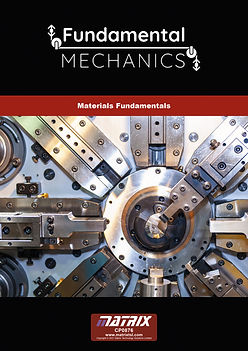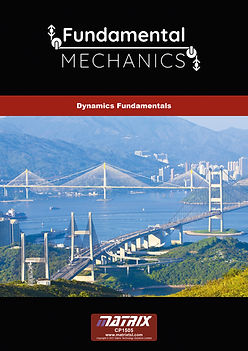top of page

Matrix TSL are proud to introduce our latest product range, Fundamental Mechanics. This range of equipment  allows students to study the principles of mechanical engineering required in many engineering related courses.

Three kits are available in the main fundamentals range,

1. Statics fundamentals

2. Materials fundamentals

3. Dynamics fundamentals## Statics fundamentals

This set of equipment covers the needs of students studying forces, moments, beams and more. Students use the storable work panel (included) to construct a range of experiments, which allow you to study a full course in static engineering systems. A full 10-hour workbook is included free of charge in the Learning Centre for this kit.

Learning objectives:

• Forces (mass, force, weight, combining, parallelogram, triangle and polygon

• Centre of gravity

• Units of weight and mass

• Free body diagrams

• Force vectors

• Coplanar forces

• Bow’s notation

• Principles of moments and moment of forces

• Distinguishing between moments and torque

• Equilibrium of forces

• Levers and the term mechanical advantage

• Simply supported beams

• Concentrated and uniform distributed loads

• Different types of pinned supports

## Statics fundamentals CurriculumStatics

## Materials fundamentals

This set of equipment covers the needs of students studying torsion, stress and strain, elastic constants, Young’s Modulus and more. Students use the storable work panel (included) to construct a range of experiments, which allow you to study a full course in material principles. A full 10-hour workbook is included free of charge in the Learning Centre for this kit.

Learning objectives:

• Torsion of rods

• What effect has Polar second moment of area on torque and modulus of rigidity

• What effect has torque, shape, length and material on rod deflection

• Tensile test using plastic, aluminium and mild steels

• Understand the terms stress and strain

• Introduction to Youngs modulus for different materials

• Terms elastic deformation and plastic deformation

• Terms yield strength and ductility

• Shear force tests

• Shear stress and shear strain

• What effect has second moment of area on beam deflection

• What effect has load, shape, length and material on beam deflection

• Different types of supports for beams

## Materials fundamentals CurriculumMaterials

## Dynamics fundamentals

This set of equipment covers the needs of students studying pulleys, static and sliding friction, mechanisms and energy conversion. Students use the storable work panel (included) to construct a range of experiments, which allow you to study a full course in dynamic engineering systems. A full 10 hour workbook is included free of charge in the Learning Centre for this kit.

Learning objectives:

• Kinetic and gravitational energy parameters and principles

• Dynamic parameters and principles

• Newton’s Law of Motion

• Flywheel experimentation

• Toggle mechanisms

• Single and compound Pulley experimentation

• static and sliding friction on Inclined planes (with frictional surfaces and rollers)

## Dynamics fundamentals CurriculumDynamics
bottom of page